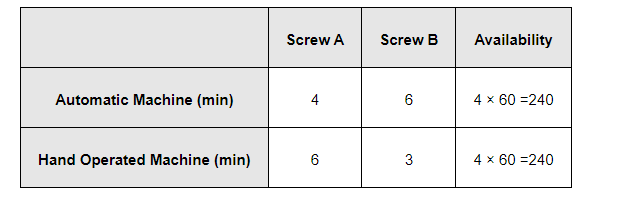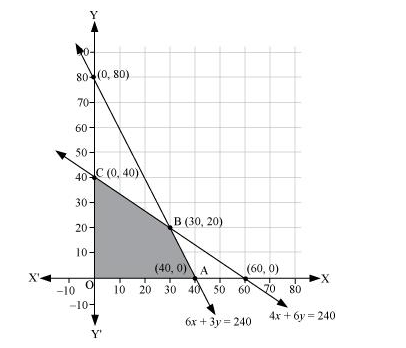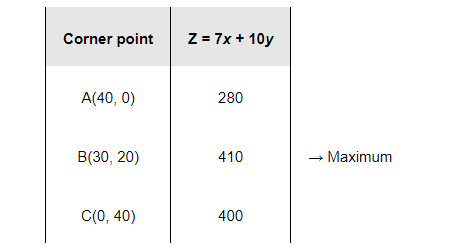# A factory manufactures two types of screws,

Question:

A factory manufactures two types of screws, A and B. Each type of screw requires the use of two machines, an automatic and a hand operated. It takes 4 minutes on the automatic and 6 minutes on hand operated machines to manufacture a package of screws A, while it takes 6 minutes on automatic and 3 minutes on the hand operated machines to manufacture a package of screws B. Each machine is available for at the most 4 hours on any day. The manufacturer can sell a package of screws A at a profit of Rs 7 and screws B at a profit of Rs10. Assuming that he can sell all the screws he manufactures, how many packages of each type should the factory owner produce in a day in order to maximize his profit? Determine the maximum profit.

Solution:

Let the factory manufacture x screws of type A and y screws of type B on each day. Therefore,

$x \geq 0$ and $y \geq 0$

The given information can be compiled in a table as follows.The profit on a package of screws A is Rs 7 and on the package of screws B is Rs 10. Therefore, the constraints are

$4 x+6 y \leq 240$

$6 x+3 y \leq 240$

Total profit, Z = 7x + 10y

The mathematical formulation of the given problem is

Maximize $Z=7 x+10 y$

subject to the constraints,

$4 x+6 y \leq 240 \ldots$ (2)

$6 x+3 y \leq 240 \quad \ldots$ (3)

$x, y \geq 0 \ldots$ (4)

The feasible region determined by the system of constraints isThe corner points are A (40, 0), B (30, 20), and C (0, 40).

The values of Z at these corner points are as follows.The maximum value of Z is 410 at (30, 20).

Thus, the factory should produce 30 packages of screws A and 20 packages of screws B to get the maximum profit of Rs 410.